• 对不同聚类结果对比实验
2019-12-03 16:20:00

数据挖掘中几种聚类算法比较：

 cluster形状 层次结构 输入参数 鲁棒性 输入数据顺序敏感 K-Means 球形 否 k 否 否 DBSCAN 任意 否 ε，MinPts 是 否 OPTICS 任意 可以 多ε，MinPts 是 否 BIRCH 任意 可以 B（扇出因子） 是 是

更多相关内容
• 几种聚类算法的对比实验 聚类方法是属于无标签的无监督学习方法。其他常见的无监督学习还有密度估计，异常检测等。聚类就是大量未知标注的数据集，按照数据的内在相似性将数据集划分为多个类别（在聚类算法中称为...

# 几种聚类算法的对比实验

聚类方法是属于无标签的无监督学习方法。其他常见的无监督学习还有密度估计，异常检测等。聚类就是对大量未知标注的数据集，按照数据的内在相似性将数据集划分为多个类别（在聚类算法中称为簇），使类别内的数据相似度高，二类别间的数据相似度低，我们可以使用聚类分析从我们的数据中获得一些有价值的见解，本文我们将研究几种常见的聚类算法，并讨论他们的优缺点。

## kmeans

from copy import deepcopy
import numpy as np
import pandas as pd
import matplotlib
matplotlib.use('TkAgg')
from matplotlib import pyplot as plt

plt.rcParams['figure.figsize'] = (16, 9)
plt.style.use('ggplot')

# 导入数据集
# print(data.shape)

# 将csv文件中的数据转换为二维数组
f1 = data['V1'].values
f2 = data['V2'].values

# 按行的方式计算两个坐标点之间的距离
def dist(a, b, ax=1):
return np.linalg.norm(a - b, axis=ax)
X = np.array(list(zip(f1, f2)))

# 设定分区数
k = 4
# 随机获得中心点的X轴坐标
C_x = np.random.randint(0, np.max(X)-20, size=k)
# 随机获得中心点的Y轴坐标
C_y = np.random.randint(0, np.max(X)-20, size=k)
C = np.array(list(zip(C_x, C_y)), dtype=np.float32)
# 用于保存中心点更新前的坐标
C_old = np.zeros(C.shape)
print(C)
# 用于保存数据所属中心点
clusters = np.zeros(len(X))
# 迭代标识位，通过计算新旧中心点的距离
iteration_flag = dist(C, C_old, 1)

fig, ax = plt.subplots()
plt.scatter(f1, f2, c='black', s=6)
ax.scatter(C[:, 0], C[:, 1], marker='*', s=200, c='blue')

tmp = 1
# 若中心点不再变化或循环次数不超过20次(此限制可取消)，则退出循环
while iteration_flag.any() != 0 and tmp < 20:
# 循环计算出每个点对应的最近中心点
for i in range(len(X)):
# 计算出每个点与中心点的距离
distances = dist(X[i], C, 1)
# print(distances)
# 记录0 - k-1个点中距离近的点
cluster = np.argmin(distances)
# 记录每个样例点与哪个中心点距离最近
clusters[i] = cluster

# 采用深拷贝将当前的中心点保存下来
# print("the distinct of clusters: ", set(clusters))
C_old = deepcopy(C)
# 从属于中心点放到一个数组中，然后按照列的方向取平均值
for i in range(k):
points = [X[j] for j in range(len(X)) if clusters[j] == i]
# print(points)
# print(np.mean(points, axis=0))
C[i] = np.mean(points, axis=0)

# 计算新旧节点的距离
print('循环第%d次' % tmp)
tmp = tmp + 1
iteration_flag = dist(C, C_old, 1)
print("新中心点与旧点的距离：", iteration_flag)

# 最终结果图示
colors = ['r', 'g', 'b', 'y', 'c', 'm']
fig, ax = plt.subplots()
# 不同的子集使用不同的颜色
for i in range(k):
points = np.array([X[j] for j in range(len(X)) if clusters[j] == i])
ax.scatter(points[:, 0], points[:, 1], s=7, c=colors[i])
ax.scatter(C[:, 0], C[:, 1], marker='*', s=200, c='black')

plt.show()



优点：

1. 简单直观，抑郁理解实现；
2. 复杂度相对比较低，在K不是很大的情况下，Kmeans的计算时间相对很短；
3. Kmean会产生紧密度比较高的簇，反映了簇内样本围绕质心的紧密程度的一种算法。
缺点：
4. 很难预测到准确的簇的数目；
5. 对初始值设置很敏感（Kmeans++）；
6. Kmeans主要发现圆形或者球形簇，对不同形状和密度的簇效果不好；

## LVQ

# -*- coding: utf-8 -*-
"""
Created on Tue Jan 29 20:22:18 2019

@author: zmddzf
"""
import numpy as np
import random
from tqdm import tqdm
import matplotlib.pyplot as plt

class LVQ:
"""
学习向量化算法实现
attributes:
train:LVQ
predict: 预测一个样本所属的簇
"""

def __init__(self, D, T, lr, maxEpoch):
"""
初始化LVQ, 构造器
:param D: 训练集, 格式为[[array, label],...]
:param T: 原型向量类别标记
:param lr: 学习率，0-1之间
:param maxEpoch: 最大迭代次数
"""
self.D = D
self.T = T
self.lr = lr
self.maxEpoch = maxEpoch
self.P = []
# 初始化原型向量，随机选取
for t in T:
while True:
p = random.choice(self.D)
if p != t:
pass
else:
self.P.append(p)
break

def __dist(self, p1, p2):
"""
私有属性，计算距离
:param p1: 向量1
:param p2: 向量2
:return dist: 距离
"""
dist = np.linalg.norm(p1 - p2)
return dist

def train(self):
"""
训练LVQ
:return self.P: 训练后的原型向量
"""
for epoch in tqdm(range(self.maxEpoch)):
x = random.choice(self.D)  # 从训练集随机选取样本
dist = []
for p in self.P:
dist.append(self.__dist(p, x))  # 计算距离列表

t = self.P[dist.index(min(dist))]  # 确定对应最小距离原型向量的类别
if t == x:
# 若类别一致, 则靠拢
self.P[dist.index(min(dist))] = self.P[dist.index(min(dist))] + self.lr * (
x - self.P[dist.index(min(dist))])
else:
# 若类别不同, 则远离
self.P[dist.index(min(dist))] = self.P[dist.index(min(dist))] - self.lr * (
x - self.P[dist.index(min(dist))])
return self.P

def predict(self, x):
"""
预测样本所属的簇
:param x: 样本向量
:return label: 样本的分类结果
"""
dist = []
for p in self.P:
dist.append(self.__dist(p, x))
label = self.P[dist.index(min(dist))]
return label

# 生成实验数据集，数据集是两个正态分布二维点集
mu1 = 2;
sigma1 = 1
mu2 = 4;
sigma2 = 1
# 生成第一个正态分布
samples1 = np.array([np.random.normal(mu1, sigma1, 50), np.random.normal(mu1, sigma1, 50)])
samples1 = samples1.T.tolist()
label1 = [1 for i in range(50)]
# 生成第二个正态分布
samples2 = np.array([np.random.normal(mu2, sigma2, 50), np.random.normal(mu2, sigma2, 50)])
samples2 = samples2.T.tolist()
label2 = [0 for i in range(50)]
# 合并生成数据集
samples = samples1 + samples2
labels = label1 + label2

# 修改数据格式
data = []
for s, l in zip(samples, labels):
data.append([np.array(s), l])

# 开始训练
lvq = LVQ(data, [0, 1], 0.1, 5000)
vector = lvq.train()

# 使用lvq分类
prediction = []
for i in data:
prediction.append(lvq.predict(i))

# 计算accuracy
accuracy = 0
for pred, label in zip(prediction, labels):
if pred == label:
accuracy += 1
accuracy = accuracy / len(data)
print("accuracy of LVQ:", accuracy)

# 画图展示原型向量和散点
plt.figure(figsize=(15, 10))
plt.scatter(np.array(samples).T, np.array(samples).T, c=labels)
plt.scatter(vector, vector, marker='*', s=300)
plt.scatter(vector, vector, marker='*', s=300)

plt.show()



LVQ算法其实也是一种基于竞争的学习，这点和无监督的SOM算法挺像的。LVD算法可以被视为一种网络，由输入层、竞争层、输出层组成。输入层很容易理解，就是接受样本的输入；竞争层可以被视为神经元之间的竞争，也就是原型向量之间的竞争，离得最近的神经元（原型向量）获胜，赢者通吃（winner-take-all）；输出层负责输出分类结果。不论是如何理解这个算法，其实本质都是一样的，也就是同类靠拢、异类远离。

## 高斯混合聚类

import matplotlib.pyplot as plt
import numpy as np
import math

# 原始数据
x = [0.697, 0.774, 0.634, 0.608, 0.556, 0.403, 0.481, 0.437, 0.666, 0.243,
0.245, 0.343, 0.639, 0.657, 0.360, 0.593, 0.719, 0.359, 0.339, 0.282,
0.748, 0.714, 0.483, 0.478, 0.525, 0.751, 0.532, 0.473, 0.725, 0.446]

y = [0.460, 0.376, 0.264, 0.318, 0.215, 0.237, 0.149, 0.211, 0.091, 0.267,
0.057, 0.099, 0.161, 0.198, 0.370, 0.042, 0.103, 0.188, 0.241, 0.257,
0.232, 0.346, 0.312, 0.437, 0.369, 0.489, 0.472, 0.376, 0.445, 0.459]

# 矩阵测试
def test_matrix():
sigma = np.mat([[0.2, 0.1], [0.0, 0.1]])
sigma_Trans = sigma.T
sigma_inverse = sigma.I
print("sigma: {}".format(sigma))
print("sigma Inverse: {}".format(sigma_inverse))
print("sigma Transform: {}".format(sigma_Trans))

def gauss_density_probability(n, x, mu, sigma):
sigma_det = np.linalg.det(sigma)
divisor = pow(2 * np.pi, n / 2) * np.sqrt(sigma_det)
exp = np.exp(-0.5 * (x - mu) * sigma.I * (x - mu).T)
p = exp / divisor
return p

# 后验概率测试
def test_posterior_probability():
xx = np.mat([[x, y]])
mu_datasets = [np.mat([[x, y]]), np.mat([[x, y]]), np.mat([[x, y]])]
sigma = np.mat([[0.1, 0.0], [0.0, 0.1]])
det = np.linalg.det(sigma)
print("det: {}".format(det))
p_all = []
for mu in mu_datasets:
p = gauss_density_probability(2, xx, mu, sigma)
p = p / 3
p_all.append(p)
p_mean = []
for p in p_all:
p_sum = np.sum(p_all)
p = p / p_sum
p_mean.append(p)
print("probability: {}".format(p_mean))

def posterior_probability(k, steps):

alpha_datasets = [np.mat([1 / k]) for _ in range(k)]
xx = [np.mat([[x[i], y[i]]]) for i in range(len(x))]
mu_rand = np.random.randint(0, 30, (1, k))
print("random: {}".format(mu_rand))
#     mu_datasets = [np.mat([[x[i], y[i]]]) for i in mu_rand]
mu_datasets = [np.mat([[x, y]]), np.mat([[x, y]]), np.mat([[x, y]])]
sigma_datasets = [np.mat([[0.1, 0.0], [0.0, 0.1]]) for _ in range(k)]
#     det = np.linalg.det(sigma_datasets)
for step in range(steps):
p_all = []
# create cluster
classification_temp = locals()
for i in range(k):
classification_temp['cluster_' + str(i)] = []
# 后验概率分组
for j in range(len(x)):
p_group = []
for i in range(k):
mu = mu_datasets[i]
p = gauss_density_probability(2, xx[j], mu, sigma_datasets[i])

p = p * alpha_datasets[i].getA()
p_group.append(p)
p_sum = np.sum(p_group)
# 取最大后验概率
max_p = max(p_group)
max_index = p_group.index(max_p)
# 最大后验概率聚类
for i in range(k):
if i == max_index:
classification_temp['cluster_' + str(max_index)].append(j)

p_group = [p_group[i] / p_sum for i in range(len(p_group))]
p_all.append(p_group)

# 更新 mu, sigma, alpha
mu_datasets = []
sigma_datasets = []
alpha_datasets = []

for i in range(k):
mu_temp_numerator = 0
mu_temp_denominator = 0
sigma_temp = 0
alpha_temp = 0
mu_numerator = [p_all[j][i] * xx[j] for j in range(len(x))]
for mm in mu_numerator:
mu_temp_numerator += mm

mu_denominator = [p_all[j][i] for j in range(len(x))]
for nn in mu_denominator:
mu_temp_denominator += nn

mu_dataset = mu_temp_numerator / mu_temp_denominator
mu_datasets.append(mu_dataset)

sigma = [p_all[j][i].getA() * (xx[j] - mu_dataset).T * (xx[j] - mu_dataset) for j in range(len(x))]
for ss in sigma:
sigma_temp += ss
sigma_dataset = sigma_temp / mu_temp_denominator
sigma_datasets.append(sigma_dataset)

alpha_new = [p_all[j][i] for j in range(len(x))]
for alpha_nn in alpha_new:
alpha_temp += alpha_nn
alpha_dataset = alpha_temp / len(x)
alpha_datasets.append(alpha_dataset)
return p_all, mu_datasets, sigma_datasets, alpha_datasets, classification_temp

def cluster_visiualization(k, steps):
post_probability, mu_datasets, sigma_datasets, alpha_datasets, classification_temp = posterior_probability(k, steps)
plt.figure(figsize=(8, 8))
markers = ['.', 's', '^', '<', '>', 'P']
plt.xlim(0.1, 0.9)
plt.ylim(0, 0.9)
plt.grid()
plt.scatter(x, y, color='r')

plt.figure(figsize=(8, 8))
for i in range(k):
# 依据聚类获取对应数据，并显示
xx = [x[num] for num in classification_temp['cluster_' + str(i)]]
yy = [y[num] for num in classification_temp['cluster_' + str(i)]]

plt.xlim(0.1, 0.9)
plt.ylim(0, 0.9)
plt.grid()
plt.scatter(xx, yy, marker=markers[i])
plt.savefig("./images/gauss_cluster.png", format="png")

if __name__ == "__main__":
cluster_visiualization(3, 100)



算法本身不复杂，可能涉及到矩阵求导的部分会麻烦一点。西瓜数据集太小了，收敛非常快。然后，这个算法同样对于初值敏感。

## DBSCAN

import numpy as np
from sklearn.cluster import DBSCAN
from sklearn import metrics
from sklearn.datasets.samples_generator import make_blobs
from sklearn.preprocessing import StandardScaler

# #############################################################################
# 产生样本数据
centers = [[1, 1], [-1, -1], [1, -1]]  # 生成聚类中心点
X, labels_true = make_blobs(n_samples=750, centers=centers, cluster_std=0.4,random_state=0) # 生成样本数据集

X = StandardScaler().fit_transform(X) # StandardScaler作用：去均值和方差归一化。且是针对每一个特征维度来做的，而不是针对样本。

# #############################################################################
# 调用密度聚类  DBSCAN
db = DBSCAN(eps=0.3, min_samples=10).fit(X)
# print(db.labels_)  # db.labels_为所有样本的聚类索引，没有聚类索引为-1
# print(db.core_sample_indices_) # 所有核心样本的索引
core_samples_mask = np.zeros_like(db.labels_, dtype=bool)  # 设置一个样本个数长度的全false向量
core_samples_mask[db.core_sample_indices_] = True #将核心样本部分设置为true
labels = db.labels_

# 获取聚类个数。（聚类结果中-1表示没有聚类为离散点）
n_clusters_ = len(set(labels)) - (1 if -1 in labels else 0)

# 模型评估
print('估计的聚类个数为: %d' % n_clusters_)
print("同质性: %0.3f" % metrics.homogeneity_score(labels_true, labels))  # 每个群集只包含单个类的成员。
print("完整性: %0.3f" % metrics.completeness_score(labels_true, labels))  # 给定类的所有成员都分配给同一个群集。
print("V-measure: %0.3f" % metrics.v_measure_score(labels_true, labels))  # 同质性和完整性的调和平均
print("调整兰德指数: %0.3f" % metrics.adjusted_rand_score(labels_true, labels))
print("调整互信息: %0.3f" % metrics.adjusted_mutual_info_score(labels_true, labels))
print("轮廓系数: %0.3f" % metrics.silhouette_score(X, labels))

# #############################################################################
# Plot result
import matplotlib.pyplot as plt

# 使用黑色标注离散点
unique_labels = set(labels)
colors = [plt.cm.Spectral(each) for each in np.linspace(0, 1, len(unique_labels))]
for k, col in zip(unique_labels, colors):
if k == -1:  # 聚类结果为-1的样本为离散点
# 使用黑色绘制离散点
col = [0, 0, 0, 1]

class_member_mask = (labels == k)  # 将所有属于该聚类的样本位置置为true

xy = X[class_member_mask & core_samples_mask]  # 将所有属于该类的核心样本取出，使用大图标绘制
plt.plot(xy[:, 0], xy[:, 1], 'o', markerfacecolor=tuple(col),markeredgecolor='k', markersize=14)

xy = X[class_member_mask & ~core_samples_mask]  # 将所有属于该类的非核心样本取出，使用小图标绘制
plt.plot(xy[:, 0], xy[:, 1], 'o', markerfacecolor=tuple(col),markeredgecolor='k', markersize=6)

plt.title('Estimated number of clusters: %d' % n_clusters_)
plt.show()


DBSCAN的主要优点有：
1） 可以对任意形状的稠密数据集进行聚类，相对的，K-Means之类的聚类算法一般只适用于凸数据集。
2） 可以在聚类的同时发现异常点，对数据集中的异常点不敏感。
3） 聚类结果没有偏倚，相对的，K-Means之类的聚类算法初始值对聚类结果有很大影响。
DBSCAN的主要缺点有：
1）如果样本集的密度不均匀、聚类间距差相差很大时，聚类质量较差，这时用DBSCAN聚类一般不适合。
2）如果样本集较大时，聚类收敛时间较长，此时可以对搜索最近邻时建立的KD树或者球树进行规模限制来改进。
3） 调参相对于传统的K-Means之类的聚类算法稍复杂，主要需要对距离阈值ϵ，邻域样本数阈值MinPts联合调参，不同的参数组合对最后的聚类效果有较大影响。

## AGNES

 #-*- coding:utf-8 -*-

import math
import pylab as pl

#数据集：每三个是一组分别是西瓜的编号，密度，含糖量
data = """
1,0.697,0.46,2,0.774,0.376,3,0.634,0.264,4,0.608,0.318,5,0.556,0.215,
6,0.403,0.237,7,0.481,0.149,8,0.437,0.211,9,0.666,0.091,10,0.243,0.267,
11,0.245,0.057,12,0.343,0.099,13,0.639,0.161,14,0.657,0.198,15,0.36,0.37,
16,0.593,0.042,17,0.719,0.103,18,0.359,0.188,19,0.339,0.241,20,0.282,0.257,
21,0.748,0.232,22,0.714,0.346,23,0.483,0.312,24,0.478,0.437,25,0.525,0.369,
26,0.751,0.489,27,0.532,0.472,28,0.473,0.376,29,0.725,0.445,30,0.446,0.459"""

#数据处理 dataset是30个样本（密度，含糖量）的列表
a = data.split(',')
dataset = [(float(a[i]), float(a[i+1])) for i in range(1, len(a)-1, 3)]

#计算欧几里得距离,a,b分别为两个元组
def dist(a, b):
return math.sqrt(math.pow(a-b, 2)+math.pow(a-b, 2))

#dist_min
def dist_min(Ci, Cj):
return min(dist(i, j) for i in Ci for j in Cj)
#dist_max
def dist_max(Ci, Cj):
return max(dist(i, j) for i in Ci for j in Cj)
#dist_avg
def dist_avg(Ci, Cj):
return sum(dist(i, j) for i in Ci for j in Cj)/(len(Ci)*len(Cj))

#找到距离最小的下标
def find_Min(M):
min = 1000
x = 0; y = 0
for i in range(len(M)):
for j in range(len(M[i])):
if i != j and M[i][j] < min:
min = M[i][j];x = i; y = j
return (x, y, min)

#算法模型：
def AGNES(dataset, dist, k):
#初始化C和M
C = [];M = []
for i in dataset:
Ci = []
Ci.append(i)
C.append(Ci)
for i in C:
Mi = []
for j in C:
Mi.append(dist(i, j))
M.append(Mi)
q = len(dataset)
#合并更新
while q > k:
x, y, min = find_Min(M)
C[x].extend(C[y])
C.remove(C[y])
M = []
for i in C:
Mi = []
for j in C:
Mi.append(dist(i, j))
M.append(Mi)
q -= 1
return C
#画图
def draw(C):
colValue = ['r', 'y', 'g', 'b', 'c', 'k', 'm']
for i in range(len(C)):
coo_X = []    #x坐标列表
coo_Y = []    #y坐标列表
for j in range(len(C[i])):
coo_X.append(C[i][j])
coo_Y.append(C[i][j])
pl.scatter(coo_X, coo_Y, marker='x', color=colValue[i%len(colValue)], label=i)

pl.legend(loc='upper right')
pl.show()

C = AGNES(dataset, dist_avg, 3)
draw(C)



AGNES算法比较简单，但一旦一组对象被合并，下一步的处理将在新生成的簇上进行。已做处理不能撤消，聚类之间也不能交换对象。增加新的样本对结果的影响较大。
假定在开始的时候有nn个簇，在结束的时候有11个簇，因此在主循环中有nn次迭代，在第ii次迭代中，我们必须在n−i+1n−i+1个簇中找到最靠近的两个进行合并。另外算法必须计算所有对象两两之间的距离，因此这个算法的复杂度为 O(n2)O(n2)，该算法对于nn很大的情况是不适用的。

展开全文机器学习
• 学 海 无 涯 一层次聚类 1层次聚类的原理及分类 1层次法Hierarchical methods先计算样本之间的距离每次将距离最近的点合并到同 一个类然后再计算类与类之间的距离将距离最近的类合并为一个大类不停的合 并直到合成了...
•python
• 机器学习实验报告5：聚类

# 〇、实验报告pdf可在该网址下载

机器学习实验五：聚类
这个需要积分下载（因为实验报告后台查重，不建议直接白嫖）。
建议看博客，博客里面会有很多实验报告小说明会用【…】加粗注释。

# 一、实验目的与要求

1. 简述K-Means聚类算法原理与算法过程。
2. 熟练掌握K-means聚类算法与结果的展示，并代码实现，做一个二维或三维空间中的2~3类点（每个类有10个点）聚类实验，把聚类结果用不同颜色与符号表示。
3. 实现人脸图像（取前23个人的人脸图像）聚类实验与旋转物体（在COIL20数据集中取前23个类的图像），把聚类结果用不同颜色与符号表示，并把对应的图像放在相应点的旁边，让人一眼看出结果对不对；同时，列表给出其在不同数据库在不同K时的聚类精度。
4. 参看前人论文，设计一个全新的聚类算法，把简要内容写在本实验报告中；把长文写好提交到 ”论文提交处“；

# 二、实验内容与方法

## 2.1 聚类算法学习与回顾

### 2.1.1 聚类任务

根据周志华老师的《机器学习》一书中，来阐述聚类任务。

#### 1）聚类任务的概念

聚类试图将数据集中的样本划分为若干个通常时不相交的子集，每个子集称为一个“簇”，通过这样的划分，每个簇可能对应于一些潜在的概念。需说明的是，这些概念对聚类算法而言事先是未知的，聚类过程仅能自动形成簇结构，簇所对应的概念语义需由使用者来把握和命名。
聚类既能作为一个单独过程，用于找寻数据内在的分布结构，也可以作为分类等其他学习任务的前驱过程。例如，在一些商业应用中需对新用户的类型进行判别，但定义“用户类型”对商家来说却可能不太容易，此时，往往可先对用户数据进行聚类，根据聚类结果将每个簇定义为一个类，然后再基于这些类训练分类模型，用于判别新用户的类型。

#### 2）符号定义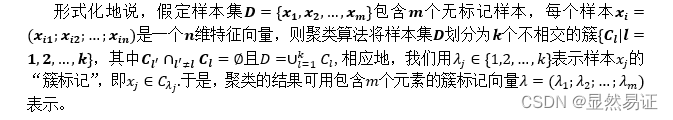#### 3）性能度量

聚类性能度量亦称为聚类“有效性指标”。监督学习中的性能度量作用相似，对聚类结果，我们需通过某种性能度量来评估其好坏；另一方面，若明确了最终将要使用的性能度量，则可以直接将其作为聚类过程的优化目标，从而更好地得到符合要求的聚类结果。
聚类是将样本集D划分为若干互不相交的自己，即样本簇，那么，什么样的聚类结果比较好呢？直观上看，我们希望“物以类聚”，即同一簇的样本尽可能彼此相似，不同簇的样本尽可能不同。换言之，聚类结果的“簇内相似度”高，且“簇间相似度”低。

聚类性能度量大致有两类，一类是将聚类结果与某个“参考模型”进行比较，称为“外部指标”；另一类是直接考察聚类结果而不利用任何参考模型，称为“内部指标”。

### 2.1.2 K-means的算法模型

#### 1）优化问题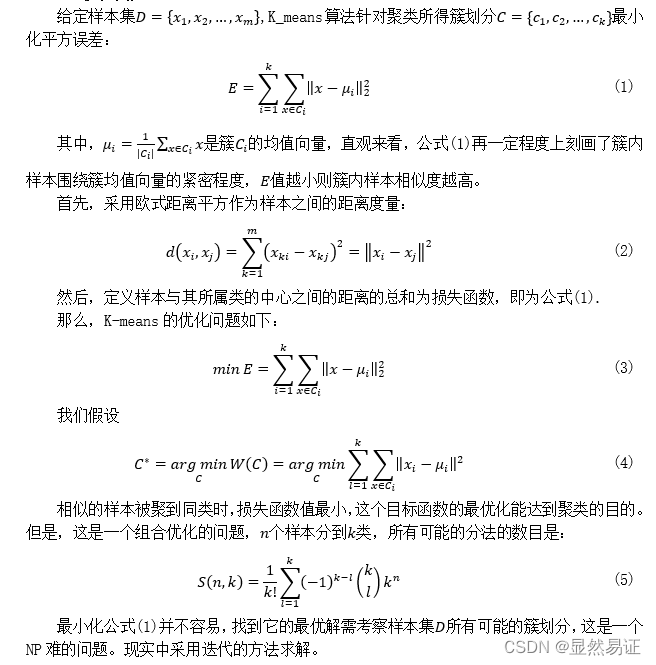#### 2）迭代策略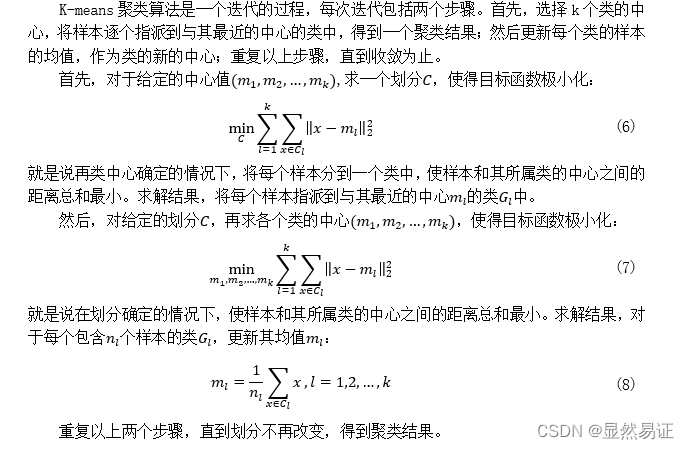### 2.1.3 K-means的算法流程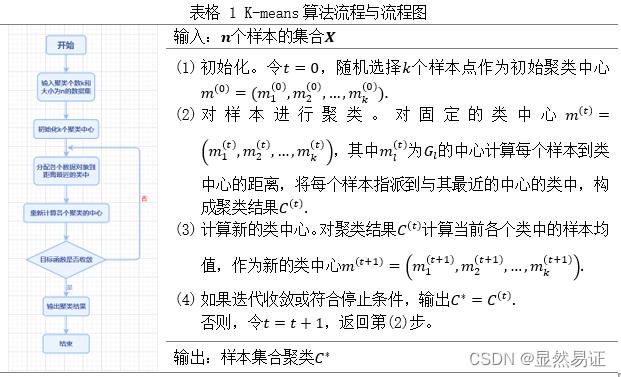### 2.1.4 K-means的算法分析

#### 1）复杂度：

K-means算法的复杂度为Ο(mnk)，其中m是样本维数，n是样本个数，k是类别个数。

#### 2）优点：

• 容易理解，聚类效果不错，虽然是局部最优， 但往往局部最优就够了；
• 处理大数据集的时候，该算法可以保证较好的伸缩性；
• 当簇近似高斯分布的时候，效果非常不错；
• 算法复杂度低。

#### 3）缺点：

• K 值需要人为设定，不同 K 值得到的结果不一样；
• 对初始的簇中心敏感，不同选取方式会得到不同结果；
• 对异常值敏感；
• 样本只能归为一类，不适合多分类任务；
• 不适合太离散的分类、样本类别不平衡的分类、非凸形状的分类。

# 三、实验步骤与过程

## 3.0 实验数据集与类标签对齐问题

### 3.0.1 数据集

（1） ORL56_46人脸数据集
该数据集共有40个人，每个人10张图片。每张图片像素大小为56×46。本次实验取该数据集下的前三个类，每个类有10个样本点。

（2） AR人脸数据集
该数据库由3个以上的数据库组成；126名受试者面部正面图像的200幅彩色图像。每个主题有26张不同的图片。对于每个受试者，这些图像被记录在两个不同的时段，间隔两周，每个时段由13张图像组成。所有图像均由同一台摄像机在严格控制的照明和视点条件下拍摄。数据库中的每个图像都是768×576像素大小，每个像素由24位RGB颜色值表示。本次实验取该数据集下的前三个类，每个类有26个样本点。

（3） FERET人脸数据集
该数据集一共200人，每人7张，已分类，灰度图，80x80像素。第1幅为标准无变化图像，第2，5幅为大幅度姿态变化图像，第3，4幅为小幅度姿态变化图像。第7幅为光照变化图像。本次实验取该数据集下的前三个类，每个类有7个样本点。

（4） COIL-20数据集
COIL-20 数据集是彩色图片集合，包含对 20 个物体从不同角度的拍摄，每隔 5 度拍摄一副图像，每个物体 72 张图像。每张图像大小进行了统一处理为 128x128。本次实验取该数据集下的前三个类，每个类有72个样本点。

### 3.0.2 类标签对齐问题和停止条件

由于本次实验牵扯到类别的判断，这个需要考虑类标签对齐的情况。这里我使用了匈牙利算法来解决聚类问题识别率计算时出现的类标签不对齐的情况，具体函数可见ACC.m.
当目标函数值的变化不超过0.01时，迭代停止。

## 3.1 二维或三维空间中的2-3类点（每个类有10个点）聚类实验

### 3.1.1 实验说明

本次实验将随机初始化一些点进行实验。每一个样本点有一个坐标和一个类标签。对于二类情况，生成20个点；针对三类情况，生成30个点。然后准确率按照聚类结果和原始类标签进行比对计算。
由于本实验的数据与ORL数据集非常类似（每个类有10个点），所以直接采用ORL数据集进行实验和可视化。实验结果见3.2.2.1.

## 3.2 人脸图像聚类实验

### 3.2.1 实验设计

本次实验以ORL56_46、AR和FERET人脸数据集进行实验。为了尽量减少由于初始值造成的结果有较大差异，每组算法我会跑五次取其平均值作为其衡量标准。

### 3.2.2 实验结果

#### 1. ORL数据集

##### 二类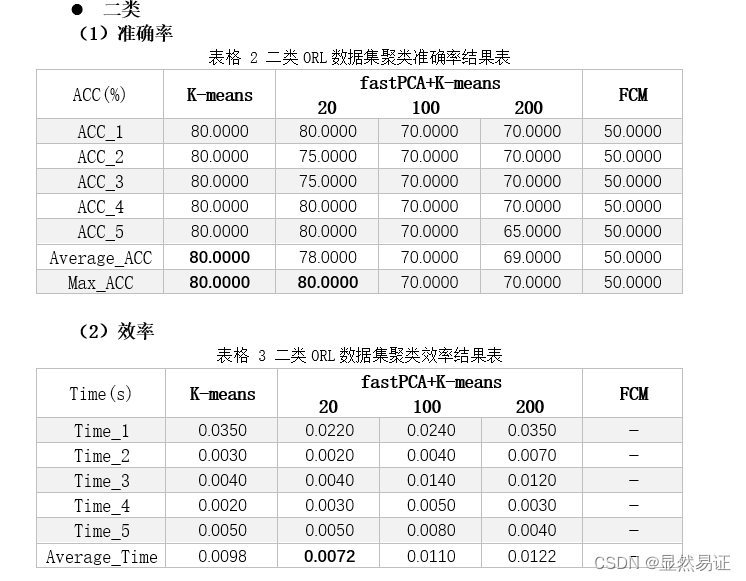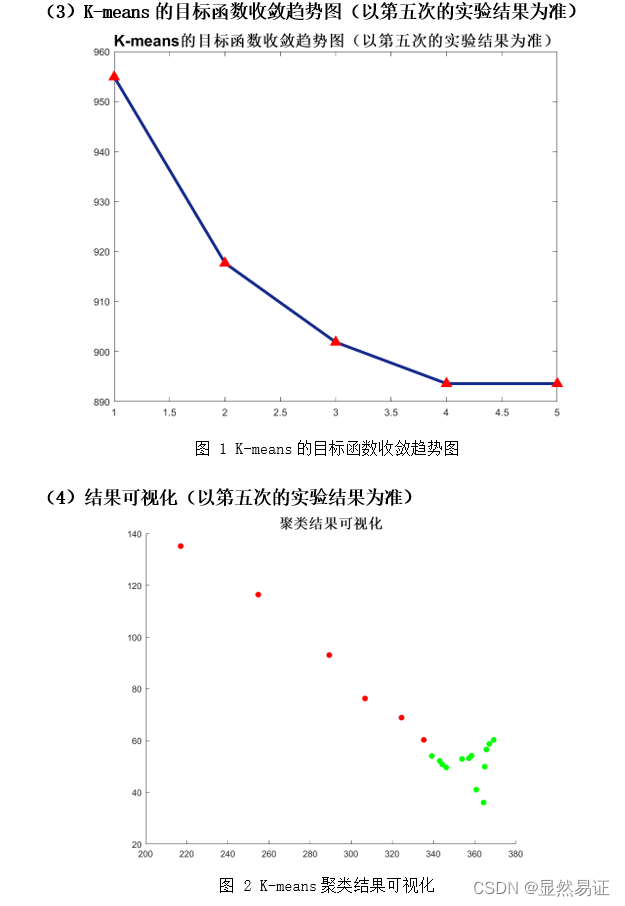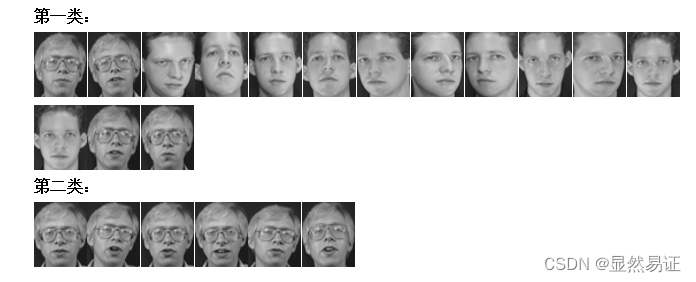##### 三类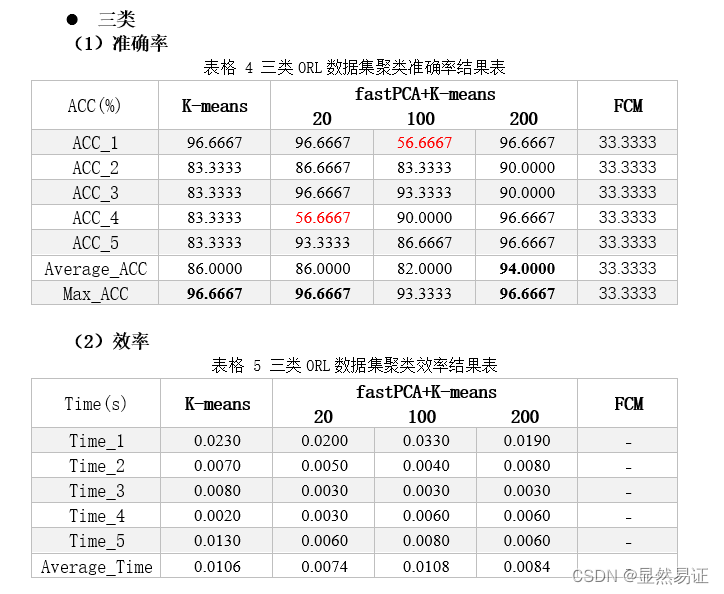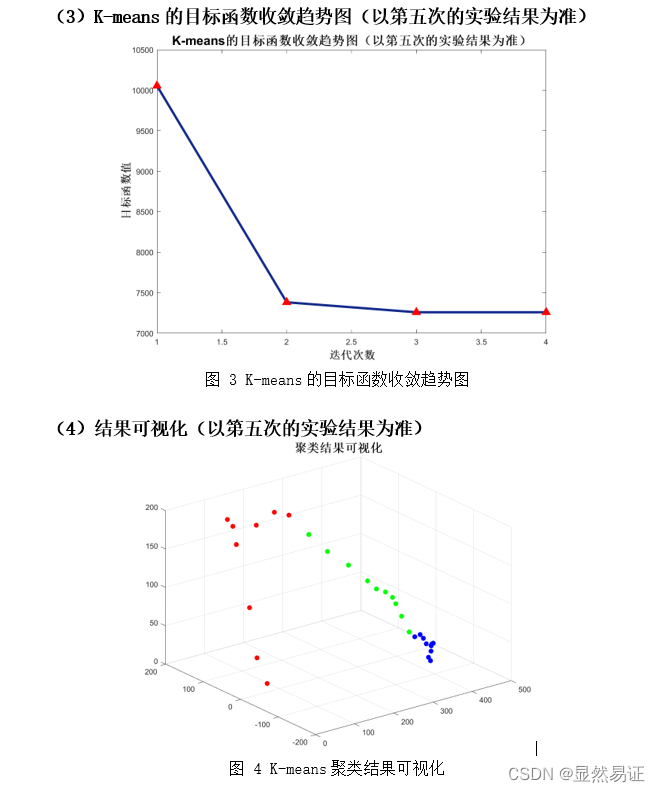#### 2. AR数据集

##### 二类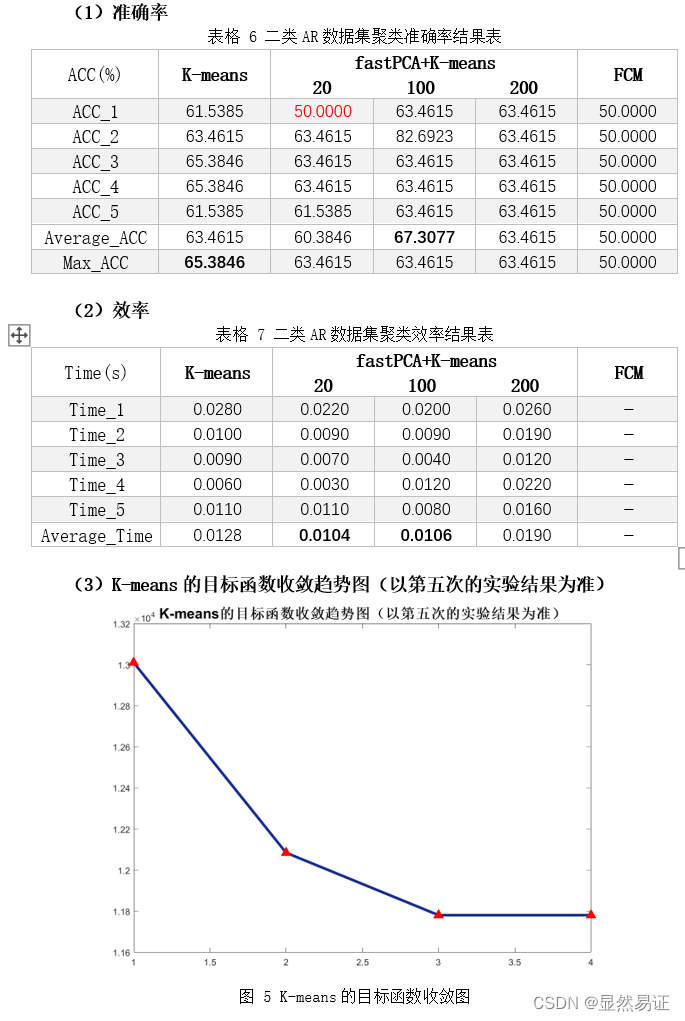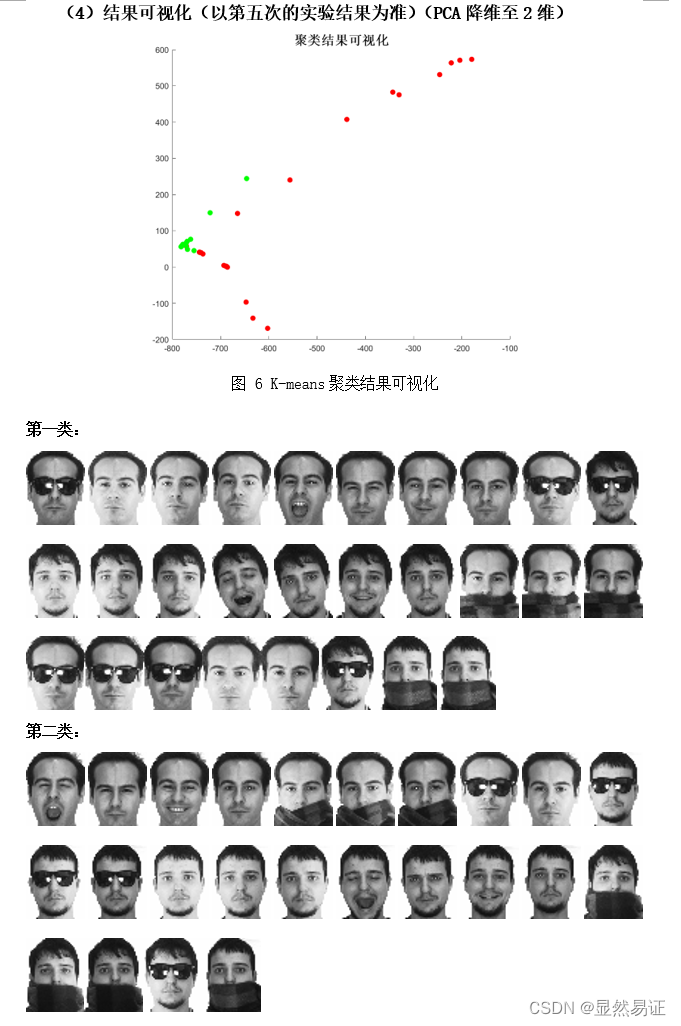##### 三类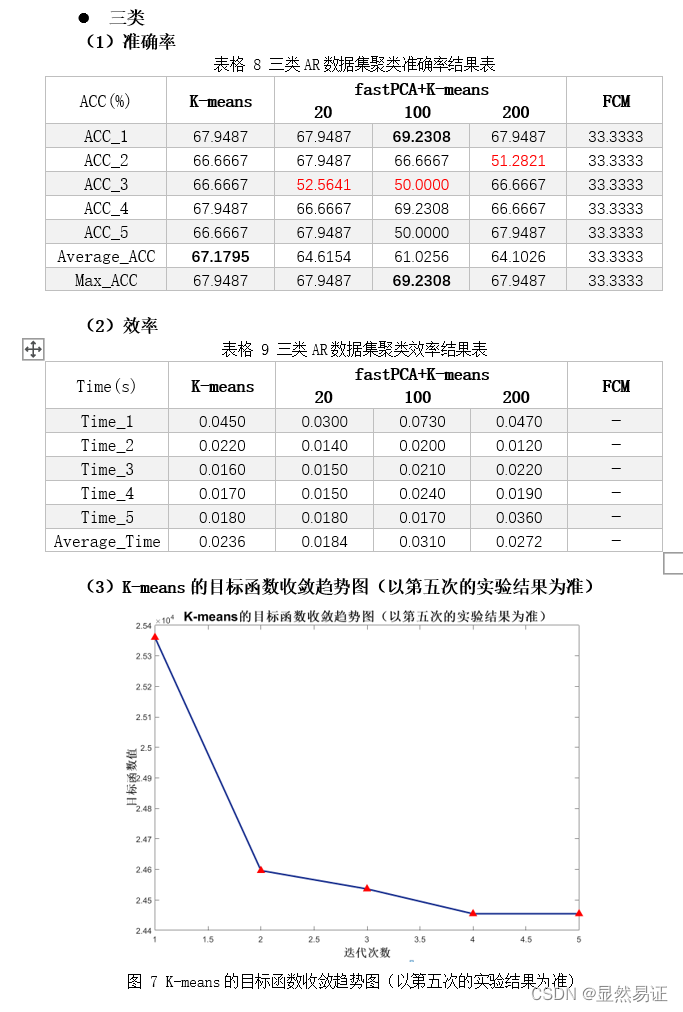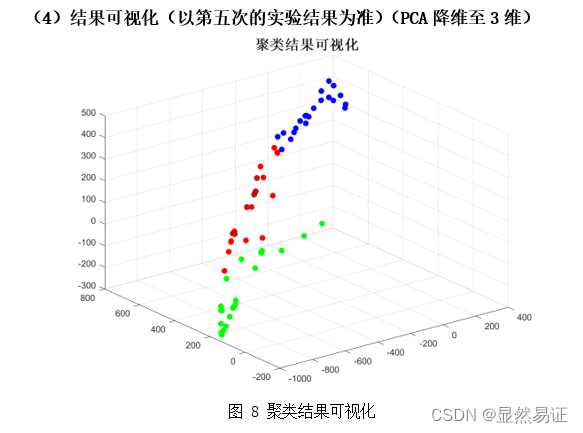#### 3. FERET数据集

##### 二类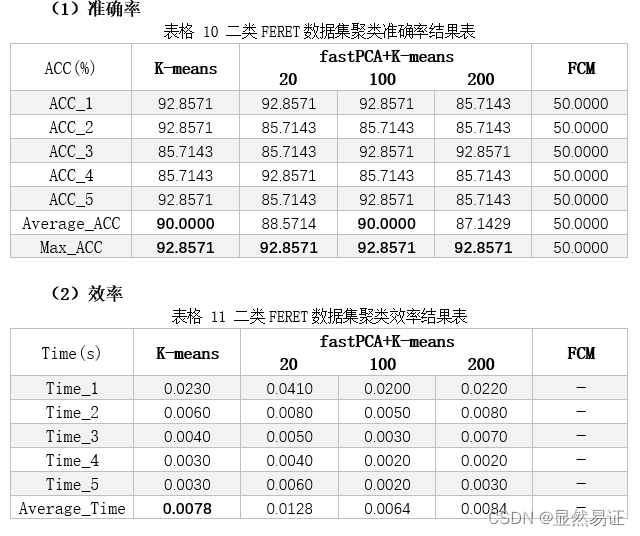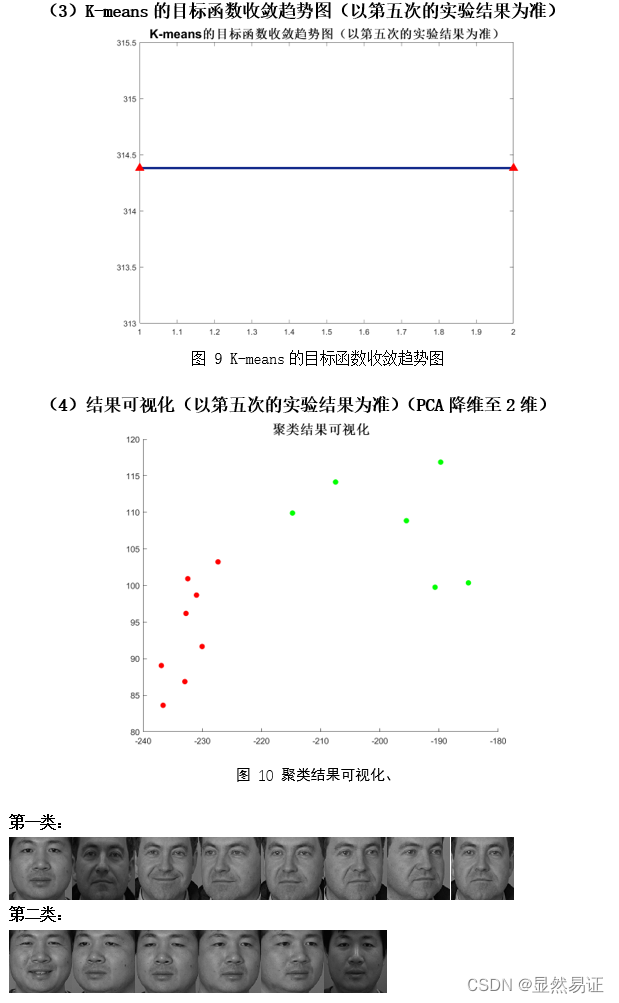##### 三类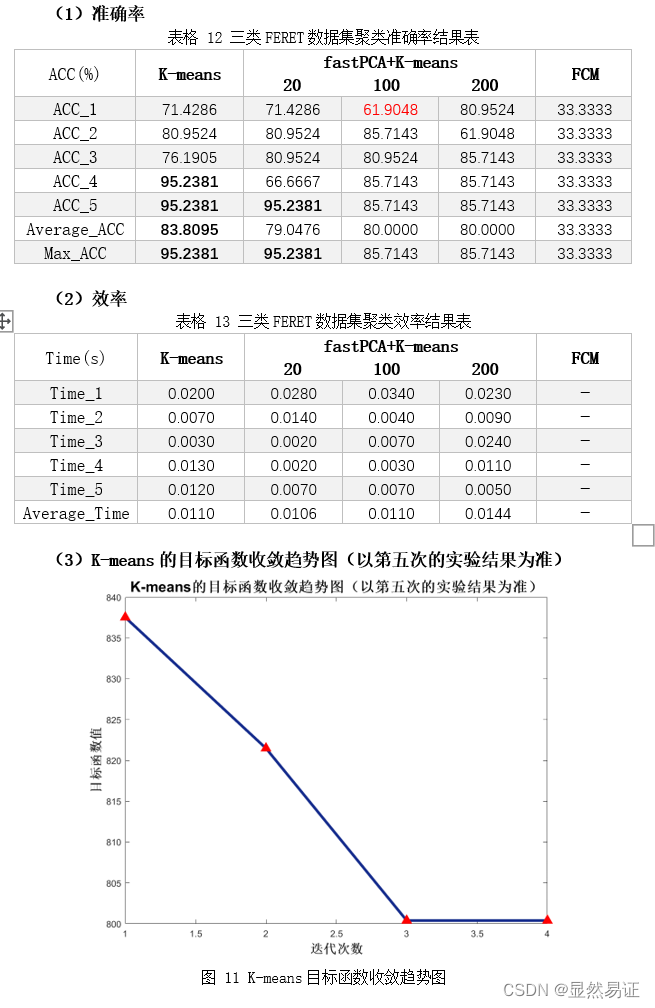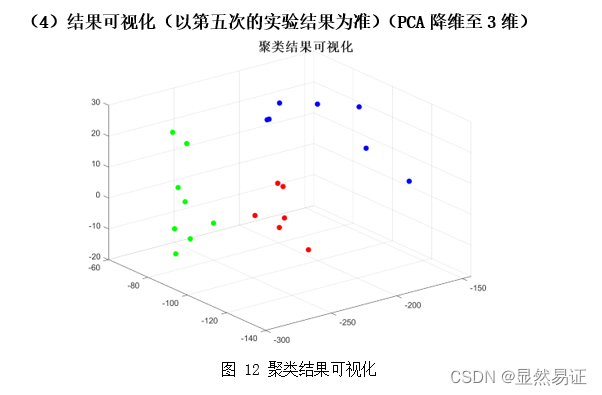### 3.2.3 实验分析

从以上三个数据集在2~3个类的聚类效果上来看，ORL的聚类效果是最好的，FERET在二类的情况较好，在三类的情况相对较差。而AR数据集在二类和三类的情况都相对较差。根据AR数据集的构成，我导出了二类情况下的AR数据集聚类结果，可以发现，K-means算法更倾向于将同一时间段的人脸照片聚集在一起，而不是按照人的类别进行聚类。相比之下，在AR数据集上进行三类的聚类效果就明显得到了改善。
因为K-means算法的聚类结果会受初始化严重影响，那么通过重复实验我们也可以得出这个结论，每次实验的准确率不是一成不变的，这也和目标函数收敛的迭代次数有关，初始化越好，迭代次数越合理，准确率越高。

## 3.3 旋转物体图像聚类实验

### 3.3.1 实验设计

本次实验采用COIL-20数据集的前2~3个类别进行实验。为了尽量减少由于初始值造成的结果有较大差异，每组算法我会跑五次取其平均值作为其衡量标准。

### 3.3.2 实验结果

##### 二类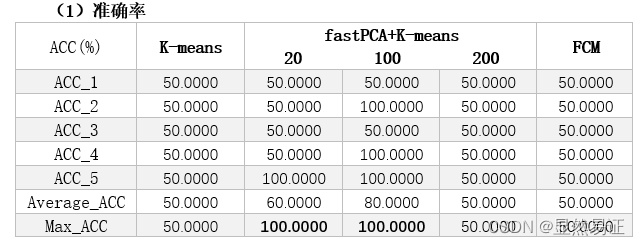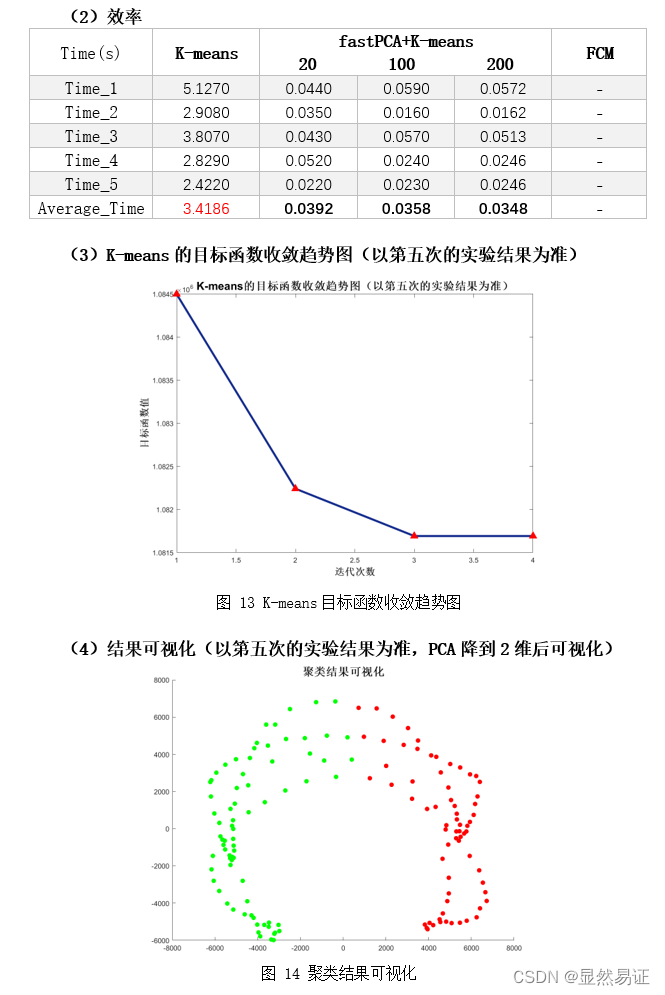##### 三类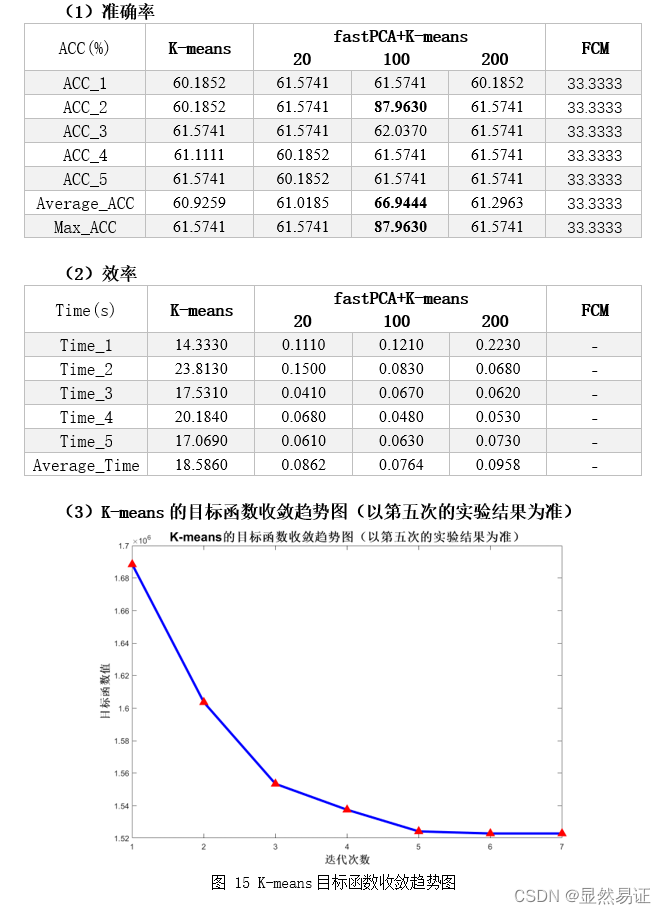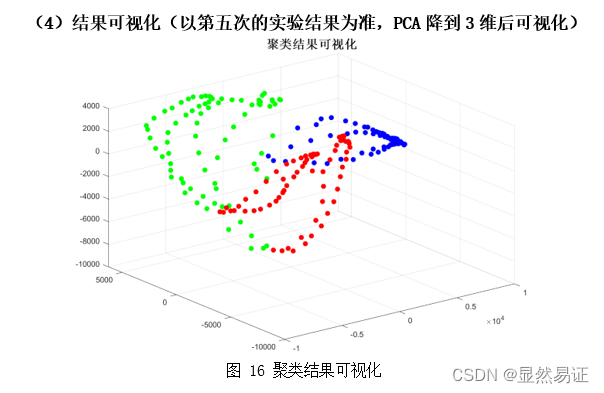### 3.3.3 实验分析

可以发现，K-means算法对旋转数据集的二类聚类效果，三类效果一般，这是因为K-means比较适用于球形数据，旋转数据在拍摄过程中会因为角度问题导致图片类内的相似度较小，所以聚类效果不佳。根据K-means的假设：K均值假设每个变量的分布是球形的；所有的变量具有相同的方差；具有相同的先验概率，要求每个类拥有相同数量的观测。可以得出以上K-means算法的缺点，单纯使用K-means算法不仅耗时长，而且聚类效果差，此时如果结合PCA降维再聚类就可以明显发现：不仅效率得到了提升、而且二类问题上识别率最高可以达到100%，效果明显改善。从另一方面可以看出初始换对聚类很重要。如果初始化的点靠的比较近，K-means会趋向于聚成一个类，导致准确率只有50%。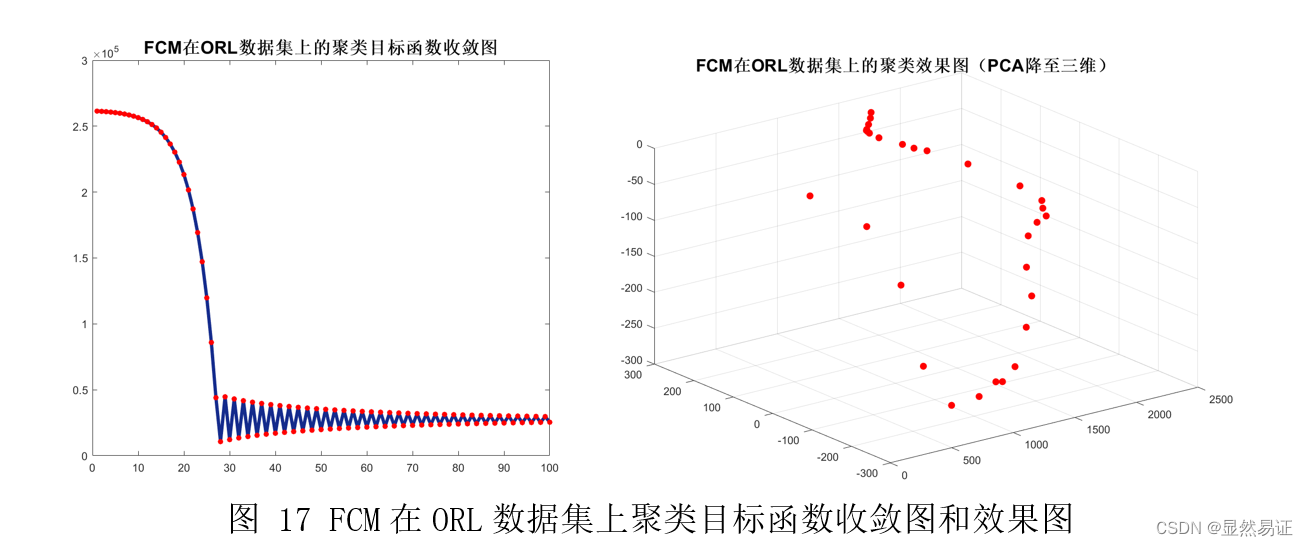根据上面两幅图，我们发现FCM在人脸聚类问题上效果并不是很好，往往FCM会因为隶属度的大小而趋向于聚成一个类，导致准确率低，进而导致准确率较低。FCM在UCI数据集上往往比K-means算法更有，一方面可能是因为维数大小不同，另一方面，人脸具有共同的特征。

【不献丑了，跳过跳过】

# 四、实验结论或体会

## 4.1 实验结论

本次实验的实验结论如下：

1. K-means算法ORL的聚类效果是最好的，FERET在二类的情况较好，在三类的情况相对较差。而AR数据集在二类和三类的情况都相对较差。根据AR数据集的构成，我导出了二类情况下的AR数据集聚类结果，可以发现，K-means算法更倾向于将同一时间段的人脸照片聚集在一起，而不是按照人的类别进行聚类。相比之下，在AR数据集上进行三类的聚类效果就明显得到了改善。因为K-means算法的聚类结果会受初始化严重影响，那么通过重复实验我们也可以得出这个结论，每次实验的准确率不是一成不变的，这也和目标函数收敛的迭代次数有关，初始化越好，迭代次数越合理，准确率越高。
2. K-means算法对旋转数据集的二类聚类效果，三类效果一般，这是因为K-means比较适用于球形数据，旋转数据在拍摄过程中会因为角度问题导致图片类内的相似度较小，所以聚类效果不佳。根据K-means的假设：K均值假设每个变量的分布是球形的；所有的变量具有相同的方差；具有相同的先验概率，要求每个类拥有相同数量的观测。可以得出以上K-means算法的缺点，单纯使用K-means算法不仅耗时长，而且聚类效果差，此时如果结合PCA降维再聚类就可以明显发现：不仅效率得到了提升、而且二类问题上识别率最高可以达到100%，效果明显改善。从另一方面可以看出初始换对聚类很重要。如果初始化的点靠的比较近，K-means会趋向于聚成一个类，导致准确率只有50%。
3. FCM在人脸聚类问题上效果并不是很好，往往FCM会因为隶属度的大小而趋向于聚成一个类，导致准确率低，进而导致准确率较低。FCM在UCI数据集上往往比K-means算法更有，一方面可能是因为维数大小不同，另一方面，猜想是因为人脸具有共同的特征，所以每个样本的隶属度会趋于一致。

## 4.2 实验体会

本次实验主要内容是聚类，这类机器学习算法相对比较容易，也是我最熟悉的机器学习模块。本次实验将K-means，fastPCA+K-means（20维，100维，200维）和FCM在人脸聚类问题上进行准确率和效率的对比。本次实验也做的比较丰富，之前也和学长一起参与过聚类算法的研究，PCM、RCM、PRFCM等一些算法也可以尝试在人脸识别的问题上做做实验。对于K-means和FCM会趋向于收敛到一个类的问题，也有专门的论文去研究此类问题，但是这种弊端在人脸聚类的任务中会更加突出。
聚类在数学建模中是一种评价类的方法，非常常用也很有意思，我也对聚类问题非常感兴趣，后期可能会继续尝试一些有关于聚类算法的研究。最后，向提出和创新算法的科研工作者致敬！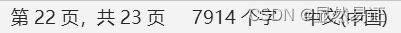展开全文机器学习 人工智能
• 0 写在前面（数据集和源代码）本文章...一共有四个代码文件，分别是Kmeans、Kmeans++、Birch和KNN算法，四个算法同一个数据集聚类分析进行对比试验。（本代码是本人自己书写，全部可用！）1 引言近年来，机器学习...

# 1 引言

近年来，机器学习已成为计算机前沿中火热的研究点之一。我国政府也将机器学习纳入了国家级战略。目前，机器学习已广泛用于各种数据挖掘、模式识别、语音识别及图像处理等各领域。本文以机器学习中四个经典的聚类算法进行对比介绍和对比实验，得出四个算法对相同实验数据集的聚类效果。

# 2聚类算法

聚类在学术界并没有一个确切的定义，此处给出1974Everitt对聚类的定义：一个类簇内的实体是相似的,不同类簇的实体是不相似的;一个类簇是测试空间中点的会聚,同一类簇的任意两个点间的距离小于不同类簇的任意两个点间的距离;类簇可以描述为一个包含密度相对较高的点集的多维空间中的连通区域,它们借助包含密度相对较低的点集的区域与其他区域(类簇)相分离。

简单地说，聚类是根据其自身特征将数据集进行划分成若干类的过程，划分的结果是相同类哈哈内数据相似度尽可能大、不同类间数据相似度尽可能小，从而发现数据集的内在结构。

聚类在不同应用领域有不同的特殊要求，聚类算法的典型性能要求有以下几个方面：

（1）伸缩性

（2）兼容性

（3）有效处理噪声数据

（4）能处理基于约束的聚类

5）可解释性和可用性

典型的聚类过程主要包括数据（样本）准备、特征选择和特征提取、相似度计算、聚类、对聚类结果进行有效性评估

聚类过程：

（1）数据准备：包括特征标准化和降维。

（2）特征选择，从最初的特征中选取最有效的特征，并将其存储于向量中。

（3）特征提取：通过对所选择的特征进行转换形成新的突出特征。

（4）聚类：首先选择合适特征类型的某种离函数 ( 或构造新的距离函数 ) 进行接近程度的度量，而后执行聚类或分组。 .

（5）聚类结果评估 : 是指对聚类结果进行评估 . 评估主要有 3 种 : 外部有效性评估、内部有效性评估和相关性测试评估。

# 3 典型聚类及其变种算法介绍

## 3.1 K-Means聚类

### 3.2.1 K-Means聚类介绍

1967 年 ,MacQueen 首次提出了 K 均值聚类算法 (K-Means 算法 )。 迄今为止 , 很多聚类任务都选择该经典算法。该算法的核心思想是找出 k个聚类中心c1 ,c2 ,…,ck使得每一个数据点 xi 和与其最近的聚类中心 cv 的平方距离和被最小化 ( 该平方距离和被称为偏差 D)。

### 3.1.2 K-means聚类算法步骤

（1）初始化，随机指定 K 个聚类中心 (c1 ,c2 ,…,ck );

（2）分配 xi, 对每一个样本 xi，找到离它最近的聚类中心 cv，并将其分配到 cv 所标明类;

（3）修正 c w，将每一个 cw 移动到其标明的类的中心；

（4）计算偏差 ；

（5）判断D是否收敛，如果D收敛，则return(c1,c2,……,ck)，并终止本算法；否则返回步骤2。

### 3.1.3 K-means 算法的优点与不足

优点: 能对大型数据集进行高效分类, 其计算复杂性为 O(tkmn), 其中 ,t 为迭代次数，k为聚类数，m 为特征属性数，n 为待分类的对象数，通常k,m,t<<n. 在对大型数据集聚类时，K-means 算法比层次聚类算法快得多。

不足: 通常会在获得一个局部最优值时终止；仅适合对数值型数据聚类；只适用于聚类。

## 3.2 K-Means++算法

### 3.2.1 K-Means++简介

由于 K-means 算法的分类结果会受到初始点的选取而有所区别，因此后来有人针对这个问题对K-Means算法进行改进，于是产生了 K-means++算法 。K-Means++算法只是对初始点的选择有改进而已，其他步骤都一样。初始质心选取的基本思路就是，初始的聚类中心之间的相互距离要尽可能的远。

### 3.2.2  K-Means++算法步骤

（1）从数据集中随机选取一个样本作为初始聚类中心c1;

（2）首先计算每个样本与当前已有聚类中心之间的最短距离（即最近的一个聚类中心的距离），用D(x)表示；接着计算每个样本被选为下一个聚类中心的概率  。按照轮盘法选出下一个聚类中心;

（3）重复第2步，直到选择出共K个聚类中心；

（4）后面与K-Means聚类算法的2-4步相同。

## 3.3 BIRCH聚类算法

### 3.3.1 BIRCH聚类简介

BIRCH的全称是利用层次方法的平衡迭代规约和聚类（Balanced Iterative Reducing and Clustering UsingHierarchies），它利用了一个类似于B+树的聚类特征树(ClusteringFeature Tree，简称CF Tree)快速的聚类。如图1所示，这颗树的每一个节点是由若干个聚类特征(Clustering Feature，简称CF)组成。每个节点包括叶子节点都有若干个CF，而内部节点的CF有指向孩子节点的指针，所有的叶子节点用一个双向链表链接起来。

不同于K-Means算法，BIRCH算法可以不用输入类别数K值。如果不输入K值，则最后的CF元组的组数即为最终的K，否则会按照输入的K值对CF元组按距离大小进行合并。一般来说，BIRCH算法适用于样本量较大的情况，除了聚类还可以额外做一些异常点检测和数据初步按类别规约的预处理。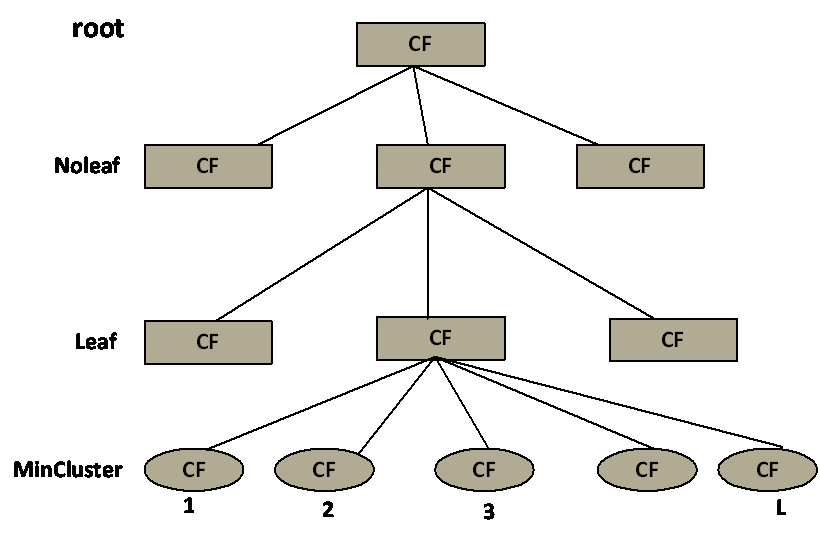图 1 CF Tree模型

3.3.2 BIRCH聚类算法步骤

（1）扫描所有数据，建立初始化的CF树，把稠密数据分成簇，稀疏数据作为孤立点对待；

（2）这个阶段是可选的，阶段（3）的全局或半全局聚类算法有着输入范围的要求，以达到速度与质量的要求，所以此阶段在阶段步骤（1）的基础上，建立一个更小的CF树；

（3）补救由于输入顺序和页面大小带来的分裂，使用全局/半全局算法对全部叶节点进行聚类

（4）这个阶段也是可选的，把阶段（3）的中心点作为种子，将数据点重新分配到最近的种子上，保证重复数据分到同一个簇中，同时添加簇标签。

### 3.3.4 BIRCH聚类的优缺点

主要优点有：

（1）节约内存，所有的样本都在磁盘上，CF Tree仅仅存了CF节点和对应的指针。

（2）聚类速度快，只需要一遍扫描训练集就可以建立CF Tree，CF Tree的增删改都很快。

（3）可以识别噪音点，还可以对数据集进行初步分类的预处理

主要缺点有：

（1）由于CF Tree对每个节点的CF个数有限制，导致聚类的结果可能和真实的类别分布不同.

（2）对高维特征的数据聚类效果不好。此时可以选择Mini Batch K-Means

（3）如果数据集的分布簇不是类似于超球体，或者说不是凸的，则聚类效果不好。

3.4 KNN聚类算法

### 3.4.1 KNN聚类简介

KNN（K-Nearest Neighbor），代表 k 个最近邻分类法，通过k个与之最相近的历史记录的组合来辨别新的记录。KNN 是一个众所周知的统计方法，在过去的 40 年里在模式识别中集中地被研究。KNN 在早期的研究策略中已被应用于文本分类，是基准 Reuters 主体的高操作性的方法之一。其它方法，如 LLSF、决策树和神经网络等。

### 3.4.2 KNN聚类算法步骤

（1）准备数据，对数据进行预处理；

（2） 选用合适的数据结构存储训练数据和测试元组；

（3）设定参数，如元组数目k；

（4）维护一个大小为k的按距离由大到小的优先级队列，用于存储最近邻训练元组。随机从训练元组中选取k个元组作为初始的最近邻元组，分别计算测试元组到这k个元组的距离，将训练元组标号和距离存入优先级队列；

（5）遍历训练元组集，计算当前训练元组与测试元组的距离，将所得距离L 与优先级队列中的最大距离Lmax；

（6）进行比较。若L>=Lmax，则舍弃该元组，遍历下一个元组。若L < Lmax，删除优先级队列中最大距离的元组，将当前训练元组存入优先级队列；

（7）遍历完毕，计算优先级队列中k 个元组的多数类，并将其作为测试元组的类别；

（8） 测试元组集测试完毕后计算误差率，继续设定不同的k值重新进行训练，最后取误差率最小的k 值。

### 3.4.3KNN聚类的优缺点

主要优点有

（1）简单，易于理解，无需建模与训练，易于实现；

（2）适合对稀有事件进行分类；

（3）适合与多分类问题，例如根据基因特征来判断其功能分类，KNN比SVM的表现要好。

主要缺点有：

（1）惰性算法，内存开销大，对测试样本分类时计算量大，性能较低；

（2）可解释性差，无法给出决策树那样的规则。

# 4 实验

### 4.1 实验环境

本实验拟将对第二节中的4个算法进行测试，实验系统采用Ubuntu16.04TLS版本（也可以用windows下pycharm等IDE），内存8G，CPU为4核心AMD芯片（两个），主频2.4GHZ。实验代码使用Python2.7.2版本。

### 4.2实验测试

为测试4个算法的聚类效果，实验将使用4个算法对同一个数据集进行聚类测试。该数据集是一个存放80个二维坐标点的文本文件，文件每行有两个数据，以制表符分割，分别表示二维坐标的X轴数据和Y轴数据。

经测试，4个算法对数据集聚类的结果图示如图2、图3、图4、图5所示：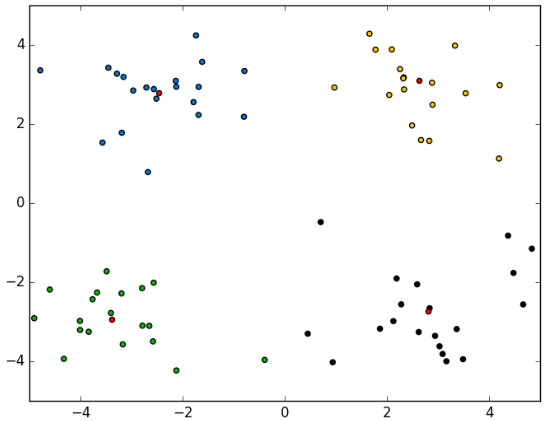图 2 K-Means聚类结果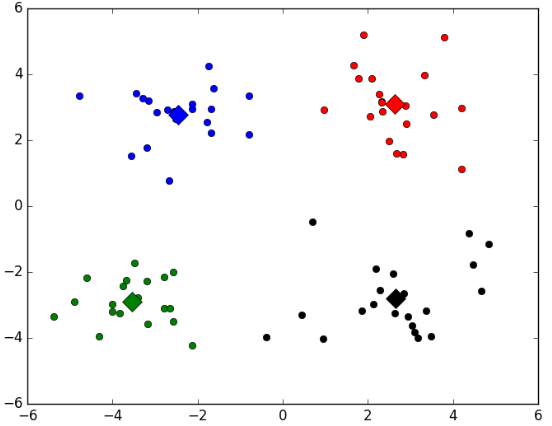图 3 K-Means++聚类结果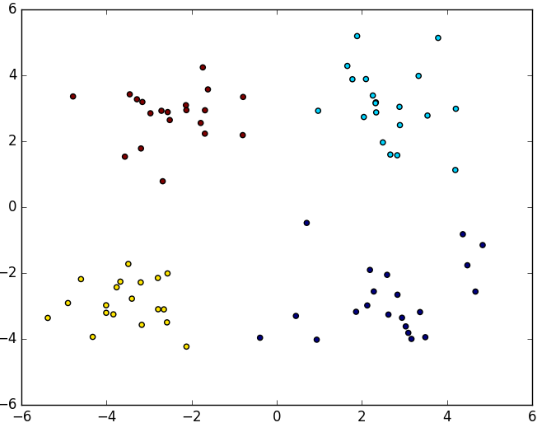图 4 BIRCH聚类结果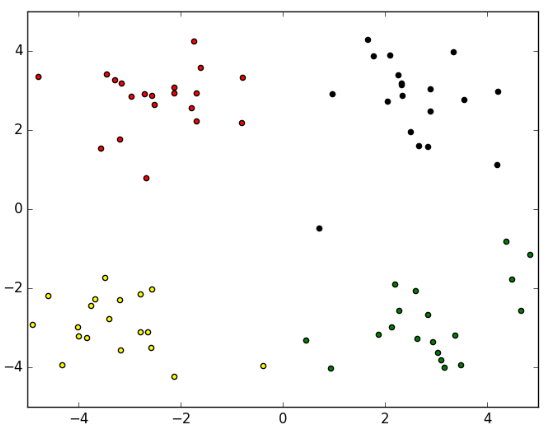图 5 KNN聚类结果

### 4.3 对比结论

上述四组图为实验对比，可以看出，四种算法对数据集的分类效果相当，只有在个别点的划分有所不同，即数据集中的(-0.392370  -3.963704)坐标点，在K-Means聚类和KNN聚类中，该点被聚类到左下方簇中，而在K-Means++聚类和BIRCH聚类中，该点被划分到了右下方簇中。

# 5总结

本文首先介绍了聚类算法定义和聚类算法的基本步骤，然后分别对K-Means、K-Means++、BIRCH和KNN四个经典的聚类算法作了详细介绍，最后通过对同一个数据集进行对比实验，得出四个聚类效果。从实验中反映了四个聚类算法对数据集的聚类效果较好。四种聚类算法在本实验中达到了的聚类效果几乎一样，但四类聚类算法在实际生活中仍将有其不同的使用背景，故在实际生活中需要结合特定的实际环境采用不同聚类算法，以达到最好的效果

# 6参考文献

 孙吉贵，刘杰，赵连宇.聚类算法研究[J].软件学报,2008

 张建萍,刘希玉.基于聚类分析的 K-means算法研究及应用[J].计算机应用研究,2007,5

 杨善林,李永森,胡笑旋,潘若愚.K-means 算法中的 k 值优化问题研究[J].系统工程理论与实践，2006,2

 David Arthur and Sergei Vassilvitskii.k-means++:[J].TheAdvantages of Careful Seeding，2007

 BIRCH:An Efficient Data 3Clustering Method forVery Large Databases[J].1996

张宁,贾自艳,史忠植.使用 KNN算法的文本分类[J].计算机工程，2005.4

KNN算法综述,https://wenku.baidu.com/view/d84cf670a5e9856a561260ce.html,2018.5.10

贺玲,吴玲达,蔡益朝.数据挖掘中的聚类算法综述[J].计算机应用研究,2007

展开全文机器学习 Kmeans聚类 Kmeans++ Birch KNN聚类
• 对比聚类 《Contrastive Clustering》 论文地址：https://www.aaai.org/AAAI21Papers/AAAI-1352.LiY.pdf 一、简介 ​ 聚类作为最基础的无监督学习算法之一，其能在不使用任何标签的情况下将样本分组到不同的簇。由于...计算机视觉 自然语言处理 表示学习
• 采用K-Means模型进行聚类，将聚类结果图示表达，用颜色区分不同簇的点，分析不同K值下的聚类结果，使用的编程语言为Python。 三、算法原理： K-Means算法的目标是将原始数据分为K簇，每一簇都有一个中心点，这也是簇...python
• 有一个实验要求对比两种大数据聚类算法的性能，具体的代码也不是由我实现的，我只是改了一部分，主要还是博客大佬们的代码，我这里借用了一下~~ 具体的实验报告和python源码文件在最后位置，提供百度云下载，本文...大数据 聚类算法 python编程
• 文章目录Contrastive Clustering文章介绍问题背景拟解决问题联合优化的应用主要贡献相关工作对比学习深度聚类实例级和聚类级的含义提出的方法模型结构PCB模块ICH模块CCH模块算法流程损失构建实验数据集介绍实验结果...python
• 1. MFCC 与距离度量方式 2. 降维和特征选择 1. 算法 1 参数对结果的影响 2. 算法 2 参数对结果的影响 3. 两种算法的对比
• 在计算机上验证和测试k-means聚类和高斯混合模型聚类实验，sklearn的相关聚类算法。 实验目的 1、掌握k-means聚类算法的原理 2、掌握高斯混合模型聚类算法的原理； 3、掌握sklearn如何实现聚类； 三、实验内容 ...matlab
• ©PaperWeekly 原创 ·作者｜李云帆学校｜四川大学博士生研究方向｜聚类，无监督表示学习论文标题：Contrastive Clustering论文来源：AAAI 2021论文链...机器学习 人工智能
• Vincent S.Tseng等人提出的基于聚类和遗传算法的时间序列分割算法中,对于适应值函数的定义存在缺陷,本文对此进行了改进:用归一化处理消除子序列幅度距离计算的影响,并引入类间距使分割结果的类间差异(模式之间的...
• 第七章实验——支持向量机题目作业一使用sklearn的DBSCAN和GaussianMixture在两个数据集上完成聚类任务；对聚类结果可视化；对比外部指标FM
• 实验五:层次聚类实验报告一、实验目的二、代码框架三、代码详解四、实验结果 一、实验目的 了解聚类的概念和层次聚类的方法 实现三种不同的层次聚类算法 对比三种不同算法在不同的数据集的情况下的性能 二、代码...
• 1.实验背景与问题提出 聚类（clustering）是无监督学习（unsuperviserd learning）中研究和应用最多的一类学习算法，目的是将样本划分成若干个“簇”（cluster），每个“簇”之间尽量相异，每个簇之内的样本尽量...python kmeans算法 数据挖掘 人工智能
• 最经典的对比学习的文章是Hinton团队提出的SimCLR，该方法首先将一个实例(图像)变换成两种不同的增广实例(图像)，然后用神经网络训练得到投影表示，用余弦相似性求出两两投影表示之间的相似性，并最大化相同实例投影...
• 文献聚类结果可视分析方法研究1 论文概述1.1 摘要1.2 引言1.3 脉络2 可视分析框架2.1 框架概述2.2 框架组成3 可视化设计3.1 语料结构可视化3.2 语料内容可视化3.3 聚类结果调整和优化4 系统实现及案例分析4.1 选择...大数据 数据挖掘
• K-means及FCM的聚类实验 算法简介 聚类算法是一种无监督学习，简单来讲就是依靠样本间聚类通过不断迭代聚类中心的方式来完成样本聚类。常见的单层聚类方式有K-means聚类和FCM聚类等。 K-means算法简介 K-means是一种...机器学习
• 随着信息时代的蓬勃发展，产生了大量多视图属性图图数据。随即，也出现许多的多视图聚类方法，但这些方法只利用数据中的多属性信息或者多拓扑图信息，没有完整地利用属性图数据的所有信息。本期 AI ...大数据 python 计算机视觉 机器学习 人工智能
• 针对套用传统的聚类方法数据流的聚类是行不通的这一问题，提出一种以遗传模拟退火算法为基础的模糊C...通过将SAGA_FCM算法和FCM算法聚类数据流的实验结果进行对比，得出采用SAGA_FCM算法聚类数据流会取得较好的效果。
• 无监督图像表示（Unsupervised image representation）显著地缩小...具体来说，SwAV 数据进行聚类的同时，加强同一图像的不同增强(或“视图”)产生的聚类结果之间的一致性，而不是像对比学习中那样直接比较特征。..机器学习
• Weka平台实现聚类算法 进一步理解聚类算法（K-平均、PAM、层次聚类、密度聚类），利用weka实现数据集的聚类处理，学会调整模型参数，以图或树的形式给出挖掘结果，并解释规则的含义。数据挖掘 机器学习
• 2.请对比sklearn的K均值聚类和混合高斯模型，针对make_blobs生成的基本数据集， makemoons生成的太极数据集，makecircles生成的圆环数据集，多类数据集，对比聚类的效果如何。 一、实验要求 在计算机上验证和测试k-...机器学习 聚类算法
• 另一方面选择一些典型的聚类算法和一些知名的数据集,主要从正确率和运行效率两个方面进行模拟实验,并分别就同一种聚类算法、不同的数据集以及同一个数据集、不同聚类算法的聚类情况进行对比分析.最后通过综合上述...
• 聚类集成是集成学习中的一个重要分支,其目标是解决无监督聚类分析中聚类算法的...文中选用了一些标准数据集,采用CEBDR算法、已有的基聚类聚类集成算法来进行对比实验,实验结果表明,该算法能够有效地提高聚类质量。...